# Make a Splash With Dynamic 2D Water EffectsSploosh! In this tutorial, I'll show you how you can use simple math, physics, and particle effects to simulate great looking 2D water waves and droplets.

Note: Although this tutorial is written using C# and XNA, you should be able to use the same techniques and concepts in almost any game development environment.

## Final Result Preview

If you have XNA, you can download the source files and compile the demo yourself. Otherwise, check out the demo video below:

There are two mostly independent parts to the water simulation.  First, we'll make the waves using a spring model.  Second, we'll use particle effects to add splashes.

## Making the Waves

To make the waves, we'll model the surface of the water as a series of vertical springs, as shown in this diagram: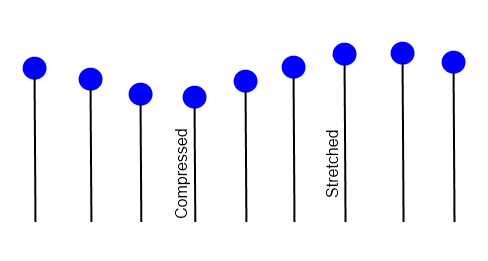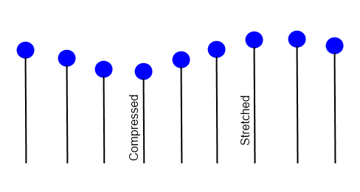This will allow the waves to bob up and down. We will then make water particles pull on their neighbouring particles to allow the waves to spread.

### Springs and Hooke's Law

One great thing about springs is that they're easy to simulate. Springs have a certain natural length; if you stretch or compress a spring, it will try to return to that natural length.

The force provided by a spring is given by Hooke's Law:

$F = -kx$

F is the force produced by the spring, k is the spring constant, and x is the spring's displacement from its natural length. The negative sign indicates the force is in the opposite direction to which the spring is displaced; if you push the spring down, it will push back up, and vice versa.

The spring constant, k, determines the stiffness of the spring.

To simulate springs, we must figure out how to move particles around based on Hooke's Law. To do this, we need a couple more formulas from physics. First, Newton's Second Law of Motion:

$F = ma$

Here, F is force, m is mass and a is acceleration. This means the stronger a force pushes on an object, and the lighter the object is, the more it accelerates.

Combining these two formulas and rearranging gives us:

$a = -\frac{k}{m} x$

This gives us the acceleration for our particles. We'll assume that all our particles will have the same mass, so we can combine k/m into a single constant.

To determine position from acceleration, we need to do numerical integration. We're going to use the simplest form of numerical integration - each frame we simply do the following:

 1 Position += Velocity;  2 Velocity += Acceleration; 

This is called the Euler method. It's not the most accurate type of numerical integration, but it's fast, simple and adequate for our purposes.

Putting it all together, our water surface particles will do the following each frame:

 1 public float Position, Velocity;  2 public void Update()  3 {  4  const float k = 0.025f; // adjust this value to your liking  5  float x = Height - TargetHeight;  6  float acceleration = -k * x;  7 8  Position += Velocity;  9  Velocity += acceleration;  10 } 

Here, TargetHeight is the natural position of the top of the spring when it's neither stretched nor compressed. You should set this value to where you want the surface of the water to be. For the demo, I set it to halfway down the screen, at 240 pixels.

### Tension and Dampening

I mentioned earlier that the spring constant, k, controls the stiffness of the spring. You can adjust this value to change the properties of the water. A low spring constant will make the springs loose. This means a force will cause large waves that oscillate slowly. Conversely, a high spring constant will increase the tension in the spring. Forces will create small waves that oscillate quickly. A high spring constant will make the water look more like jiggling Jello.

A word of warning: do not set the spring constant too high. Very stiff springs apply very strong forces that change greatly in a very small amount of time. This does not play well with numerical integration, which simulates the springs as a series of discrete jumps at regular time intervals. A very stiff spring can even have an oscillation period that's shorter than your time step. Even worse, the Euler method of integration tends to gain energy as the simulation becomes less accurate, causing stiff springs to explode.

There is a problem with our spring model so far. Once a spring starts oscillating, it will never stop. To solve this we must apply some dampening. The idea is to apply a force in the opposite direction that our spring is moving in order to slow it down. This requires a small adjustment to our spring formula:

$a = -\frac{k}{m} x - dv$

Here, v is velocity and d is the dampening factor - another constant you can tweak to adjust the feel of the water. It should be fairly small if you want your waves to oscillate. The demo uses a dampening factor of 0.025. A high dampening factor will make the water look thick like molasses, while a low value will allow the waves to oscillate for a long time.

### Making the Waves Propagate

Now that we can make a spring, let's use them to model water. As shown in the first diagram, we're modelling the water using a series of parallel, vertical springs. Of course, if the springs are all independent, the waves will never spread out like real waves do.

I'll show the code first, and then go over it:

 1 for (int i = 0; i < springs.Length; i++)  2  springs[i].Update(Dampening, Tension);  3 4 float[] leftDeltas = new float[springs.Length];  5 float[] rightDeltas = new float[springs.Length];  6   7 // do some passes where springs pull on their neighbours  8 for (int j = 0; j < 8; j++)  9 {  10  for (int i = 0; i < springs.Length; i++)  11  {  12  if (i > 0)  13  {  14  leftDeltas[i] = Spread * (springs[i].Height - springs [i - 1].Height);  15  springs[i - 1].Speed += leftDeltas[i];  16  }  17  if (i < springs.Length - 1)  18  {  19  rightDeltas[i] = Spread * (springs[i].Height - springs [i + 1].Height);  20  springs[i + 1].Speed += rightDeltas[i];  21  }  22  }  23 24  for (int i = 0; i < springs.Length; i++)  25  {  26  if (i > 0)  27  springs[i - 1].Height += leftDeltas[i];  28  if (i < springs.Length - 1)  29  springs[i + 1].Height += rightDeltas[i];  30  }  31 } 

This code would be called every frame from your Update() method. Here, springs is an array of springs, laid out from left to right. leftDeltas is an array of floats that stores the difference in height between each spring and its left neighbour. rightDeltas is the equivalent for the right neighbours. We store all these height differences in arrays because the last two if statements modify the heights of the springs. We have to measure the height differences before any of the heights are modified.

The code starts by running Hooke's Law on each spring as described earlier. It then looks at the height difference between each spring and its neighbours, and each spring pulls its neighbouring springs towards itself by altering the neighbours' positions and velocities. The neighbour-pulling step is repeated eight times to allow the waves to propagate faster.

There's one more tweakable value here called Spread. It controls how fast the waves spread. It can take values between 0 and 0.5, with larger values making the waves spread out faster.

To start the waves moving, we're going to add a simple method called Splash().

 1 public void Splash(int index, float speed)  2 {  3  if (index >= 0 && index < springs.Length)  4  springs[i].Speed = speed;  5 } 

Any time you want to make waves, call Splash(). The index parameter determines at which spring the splash should originate, and the speed parameter determines how large the waves will be.

### Rendering

We'll be using the XNA PrimitiveBatch class from the XNA PrimitivesSample. The PrimitiveBatch class helps us draw lines and triangles directly with the GPU. You use it like so:

 1 // in LoadContent()  2 primitiveBatch = new PrimitiveBatch(GraphicsDevice);  3 4 // in Draw()  5 primitiveBatch.Begin(PrimitiveType.TriangleList);  6 foreach (Triangle triangle in trianglesToDraw)  7 {  8  primitiveBatch.AddVertex(triangle.Point1, Color.Red);  9  primitiveBatch.AddVertex(triangle.Point2, Color.Red);  10  primitiveBatch.AddVertex(triangle.Point3, Color.Red);  11 }  12 primitiveBatch.End(); 

One thing to note is that, by default, you must specify the triangle vertices in a clockwise order. If you add them in a counter clockwise order the triangle will be culled and you won't see it.

It's not necessary to have a spring for each pixel of width. In the demo I used 201 springs spread across an 800 pixel wide window. That gives exactly 4 pixels between each spring, with the first spring at 0 and the last at 800 pixels. You could probably use even fewer springs and still have the water look smooth.

What we want to do is draw thin, tall trapezoids that extend from the bottom of the screen to the surface of the water and connect the springs, as shown in this diagram: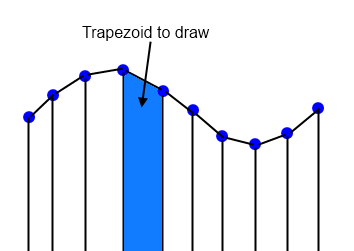Since graphics cards don't draw trapezoids directly, we have to draw each trapezoid as two triangles. To make it look a bit nicer, we'll also make the water darker as it gets deeper by colouring the bottom vertices dark blue. The GPU will automatically interpolate colours between the vertices.

 1 primitiveBatch.Begin(PrimitiveType.TriangleList);  2 Color midnightBlue = new Color(0, 15, 40) * 0.9f;  3 Color lightBlue = new Color(0.2f, 0.5f, 1f) * 0.8f;  4 5 var viewport = GraphicsDevice.Viewport;  6 float bottom = viewport.Height;  7 8 // stretch the springs' x positions to take up the entire window  9 float scale = viewport.Width / (springs.Length - 1f); // be sure to use float division  10 11 for (int i = 1; i < springs.Length; i++)  12 {  13  // create the four corners of our triangle.  14  Vector2 p1 = new Vector2((i - 1) * scale, springs[i - 1].Height);  15  Vector2 p2 = new Vector2(i * scale, springs[i].Height);  16  Vector2 p3 = new Vector2(p2.X, bottom);  17  Vector2 p4 = new Vector2(p1.X, bottom);  18 19  primitiveBatch.AddVertex(p1, lightBlue);  20  primitiveBatch.AddVertex(p2, lightBlue);  21  primitiveBatch.AddVertex(p3, midnightBlue);  22 23  primitiveBatch.AddVertex(p1, lightBlue);  24  primitiveBatch.AddVertex(p3, midnightBlue);  25  primitiveBatch.AddVertex(p4, midnightBlue);  26 }  27 28 primitiveBatch.End(); 

Here is the result:

## Making the Splashes

The waves look pretty good, but I'd like to see a splash when the rock hits the water. Particle effects are perfect for this.

### Particle Effects

A particle effect uses a large number of small particles to produce some visual effect. They're sometimes used for things like smoke or sparks. We're going to use particles for the water droplets in the splashes.

The first thing we need is our particle class:

 1 class Particle  2 {  3  public Vector2 Position;  4  public Vector2 Velocity;  5  public float Orientation;  6 7  public Particle(Vector2 position, Vector2 velocity, float orientation)  8  {  9  Position = position;  10  Velocity = velocity;  11  Orientation = orientation;  12  }  13 } 

This class just holds the properties a particle can have. Next, we create a list of particles.

 1 List particles = new List(); 

Each frame, we must update and draw the particles.

 1 void UpdateParticle(Particle particle)  2 {  3  const float Gravity = 0.3f;  4  particle.Velocity.Y += Gravity;  5  particle.Position += particle.Velocity;  6  particle.Orientation = GetAngle(particle.Velocity);  7 }  8 9 private float GetAngle(Vector2 vector)  10 {  11  return (float)Math.Atan2(vector.Y, vector.X);  12 }  13 14 public void Update()  15 {  16  foreach (var particle in particles)  17  UpdateParticle(particle);  18 19  // delete particles that are off-screen or under water  20  particles = particles.Where(x => x.Position.X >= 0 && x.Position.X <= 800 && x.Position.Y <= GetHeight(x.Position.X)).ToList();  21 } 

We update the particles to fall under gravity and set the particle's orientation to match the direction it's going in. We then get rid of any particles that are off-screen or under water by copying all the particles we want to keep into a new list and assigning it to particles. Next we draw the particles.

 1 void DrawParticle(Particle particle)  2 {  3  Vector2 origin = new Vector2(ParticleImage.Width, ParticleImage.Height) / 2f;  4  spriteBatch.Draw(ParticleImage, particle.Position, null, Color.White, particle.Orientation, origin, 0.6f, 0, 0);  5 }  6 7 public void Draw()  8 {  9  foreach (var particle in particles)  10  DrawParticle(particle);  11 } 

Below is the texture I used for the particles.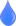Now, whenever we create a splash, we make a bunch of particles.

 1 private void CreateSplashParticles(float xPosition, float speed)  2 {  3  float y = GetHeight(xPosition);  4 5  if (speed > 60)  6  {  7  for (int i = 0; i < speed / 8; i++)  8  {  9  Vector2 pos = new Vector2(xPosition, y) + GetRandomVector2(40);  10  Vector2 vel = FromPolar(MathHelper.ToRadians(GetRandomFloat(-150, -30)), GetRandomFloat(0, 0.5f * (float)Math.Sqrt(speed)));  11  particles.Add(new Particle(pos, velocity, 0));  12  }  13  }  14 } 

You can call this method from the Splash() method we use to make waves. The parameter speed is how fast the rock hits the water. We'll make bigger splashes if the rock is moving faster.

GetRandomVector2(40) returns a vector with a random direction and a random length between 0 and 40. We want to add a little randomness to the positions so the particles don't all appear at a single point. FromPolar() returns a Vector2 with a given direction and length.

Here is the result:

### Using Metaballs as Particles

Our splashes look pretty decent, and some great games, like World of Goo, have particle effect splashes that look much like ours. However, I'm going to show you a technique to make the splashes look more liquid-like. The technique is using metaballs, organic-looking blobs which I've written a tutorial about before. If you're interested in the details about metaballs and how they work, read that tutorial. If you just want to know how to apply them to our splashes, keep reading.

Metaballs look liquid-like in the way they fuse together, making them a good match for our liquid splashes. To make the metaballs, we will need to add new class variables:

 1 RenderTarget2D metaballTarget;  2 AlphaTestEffect alphaTest; 

Which we initialize like so:

 1 var view = GraphicsDevice.Viewport;  2 metaballTarget = new RenderTarget2D(GraphicsDevice, view.Width, view.Height);  3 alphaTest = new AlphaTestEffect(GraphicsDevice);  4 alphaTest.ReferenceAlpha = 175;  5   6 alphaTest.Projection = Matrix.CreateTranslation(-0.5f, -0.5f, 0) *  7  Matrix.CreateOrthographicOffCenter(0, view.Width, view.Height, 0, 0, 1); 

Then we draw the metaballs:

 1 GraphicsDevice.SetRenderTarget(metaballTarget);  2 GraphicsDevice.Clear(Color.Transparent);  3 4 Color lightBlue = new Color(0.2f, 0.5f, 1f);  5 6 spriteBatch.Begin(0, BlendState.Additive);  7 foreach (var particle in particles)  8 {  9  Vector2 origin = new Vector2(ParticleImage.Width, ParticleImage.Height) / 2f;  10  spriteBatch.Draw(ParticleImage, particle.Position, null, lightBlue, particle.Orientation, origin, 2f, 0, 0);  11 }  12 spriteBatch.End();  13 14 GraphicsDevice.SetRenderTarget(null);  15 device.Clear(Color.CornflowerBlue);  16 spriteBatch.Begin(0, null, null, null, null, alphaTest);  17 spriteBatch.Draw(metaballTarget, Vector2.Zero, Color.White);  18 spriteBatch.End();  19 20 // draw waves and other things 

The metaball effect depends on having a particle texture that fades out as you get further from the center. Here's what I used, set on a black background to make it visible: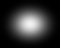Here's what it looks like:

The water droplets now fuse together when they are close. However, they don't fuse with the surface of the water. We can fix this by adding a gradient to the water's surface that makes it gradually fade out, and rendering it to our metaball render target.

Add the following code to the above method before the line GraphicsDevice.SetRendertarget(null):

 1 primitiveBatch.Begin(PrimitiveType.TriangleList);  2 3 const float thickness = 20;  4 float scale = GraphicsDevice.Viewport.Width / (springs.Length - 1f);  5 for (int i = 1; i < springs.Length; i++)  6 {  7  Vector2 p1 = new Vector2((i - 1) * scale, springs[i - 1].Height);  8  Vector2 p2 = new Vector2(i * scale, springs[i].Height);  9  Vector2 p3 = new Vector2(p1.X, p1.Y - thickness);  10  Vector2 p4 = new Vector2(p2.X, p2.Y - thickness);  11 12  primitiveBatch.AddVertex(p2, lightBlue);  13  primitiveBatch.AddVertex(p1, lightBlue);  14  primitiveBatch.AddVertex(p3, Color.Transparent);  15 16  primitiveBatch.AddVertex(p3, Color.Transparent);  17  primitiveBatch.AddVertex(p4, Color.Transparent);  18  primitiveBatch.AddVertex(p2, lightBlue);  19 }  20 21 primitiveBatch.End(); 

Now the particles will fuse with the water's surface.

The water particles look a bit flat, and it would be nice to give them some shading. Ideally, you would do this in a shader. However, for the sake of keeping this tutorial simple, we're going to use a quick and easy trick: we're simply going to draw the particles three times with different tinting and offsets, as illustrated in the diagram below.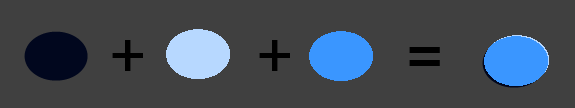To do this, we want to capture the metaball particles in a new render target. We'll then draw that render target once for each tint.

First, declare a new RenderTarget2D just like we did for the metaballs:

 1 particlesTarget = new RenderTarget2D(GraphicsDevice, view.Width, view.Height); 

Then, instead of drawing metaballsTarget directly to the backbuffer, we want to draw it onto particlesTarget. To do this, go to the method where we draw the metaballs and simply change these lines:

 1 GraphicsDevice.SetRenderTarget(null);  2 device.Clear(Color.CornflowerBlue); 

...to:

 1 GraphicsDevice.SetRenderTarget(particlesTarget);  2 device.Clear(Color.Transparent); 

Then use the following code to draw the particles three times with different tints and offsets:

 1 Color lightBlue = new Color(0.2f, 0.5f, 1f);  2 3 GraphicsDevice.SetRenderTarget(null);  4 device.Clear(Color.CornflowerBlue);  5 6 spriteBatch.Begin();  7 spriteBatch.Draw(particlesTarget, -Vector2.One, new Color(0.8f, 0.8f, 1f));  8 spriteBatch.Draw(particlesTarget, Vector2.One, new Color(0f, 0f, 0.2f));  9 spriteBatch.Draw(particlesTarget, Vector2.Zero, lightBlue);  10 spriteBatch.End();  11 12 // draw waves and other stuff 

## Conclusion

That's it for basic 2D water. For the demo, I added a rock you can drop into the water. I draw the water with some transparency on top of the rock to make it look like it's underwater, and make it slow down when it's underwater due to water resistance.

To make the demo look a bit nicer, I went to opengameart.org and found an image for the rock and a sky background. You can find the rock and sky at http://opengameart.org/content/rocks and opengameart.org/content/sky-backdrop respectively.